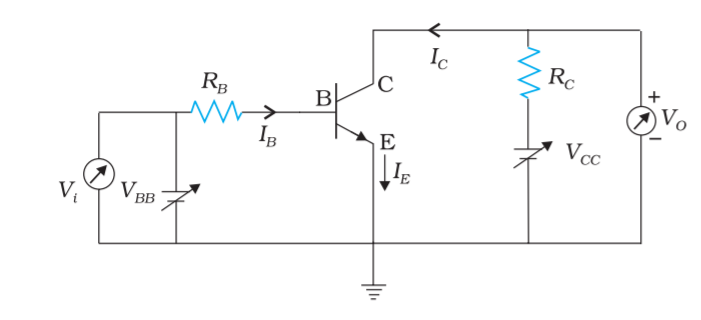Home/Class 12/Physics/

## QuestionPhysicsClass 12

In figure , the   $$V_{\mathit{BB}}$$ supply can be varied from $$0 \;V$$ to $$5.0\;V$$ . The Si transistor has $$\beta _{\mathit{dc}}=250$$  and $$R_B=100\;k\Omega ,R_C=1\;KV_{\mathit{CC}}=5.0\;V$$  Assume that when the transistor is saturated, $$V_{\mathit{CE}}=0\;V$$  and $$V_{\mathit{BE}}=0.8\;\mathit{V.}$$  Calculate the minimum base current, for which the transistor will reach saturation. Hence, determine $$V{_1}$$ , when the transistor is switched on.Given at saturation $$V_{\mathit{CE}}=0\;V,V_{\mathit{BE}}=0.8\;V$$
$$V_{\mathit{CE}}=V_{\mathit{CC}}-I_CR_C$$
$$I_C=\frac{V_{\mathit{CC}}}{R_C}=\frac{5.0\;V}{1.0\;k\Omega} =5.0\;\mathit{mA}$$
Therefore $$I_B=\frac{I_C}{\beta }=\frac{5.0\;\mathit{mA}}{250}=20\mu A$$
The input voltage at which the transistor will go into saturation is given by $$V_{\mathit{IH}}=V_{\mathit{BB}}=I_BR_B+V_{\mathit{BE}}$$
$$=20\mu A\times 100k\Omega +0.8V=2.8V$$